# ACTIVE_FILTERS.pptx

31 de May de 2023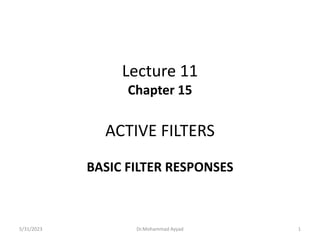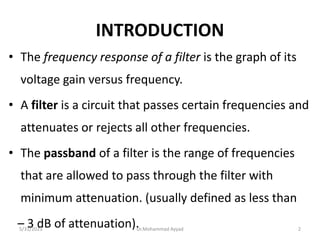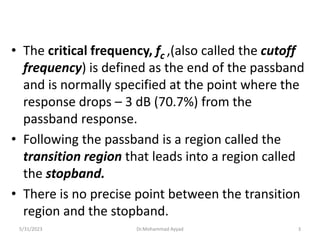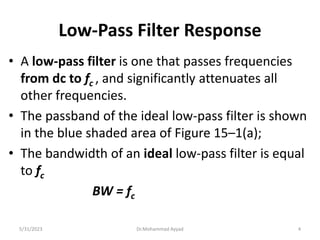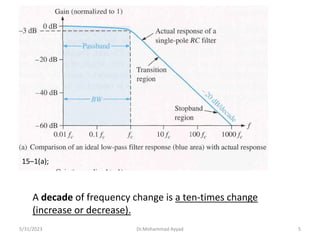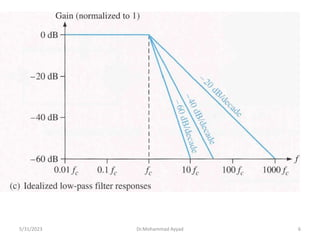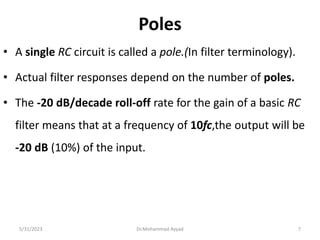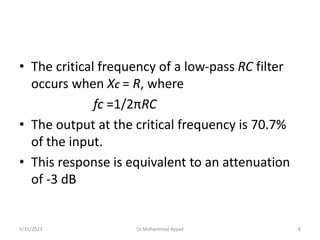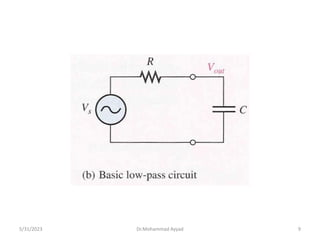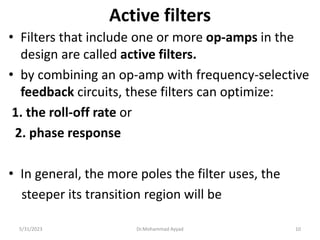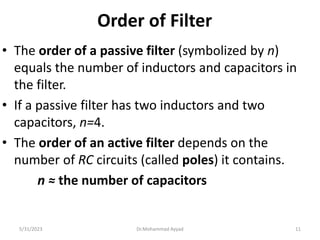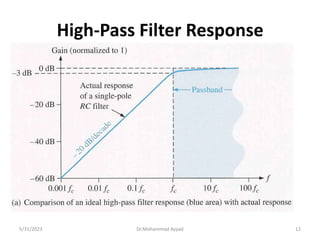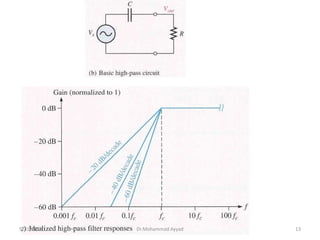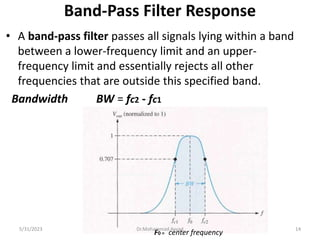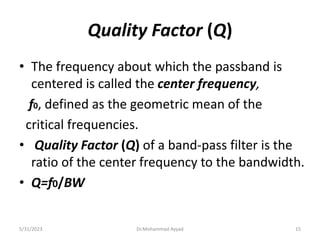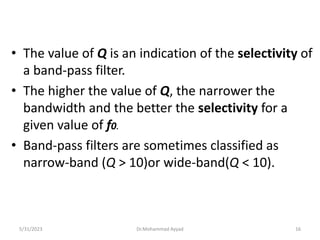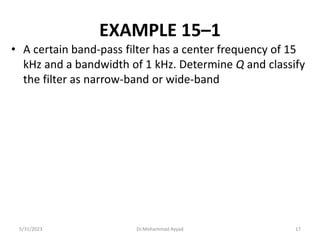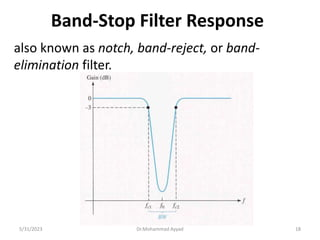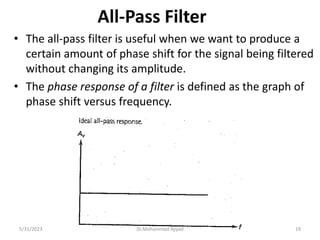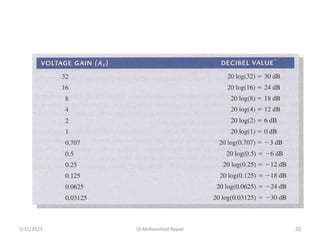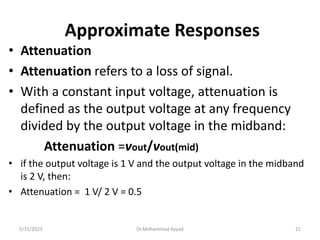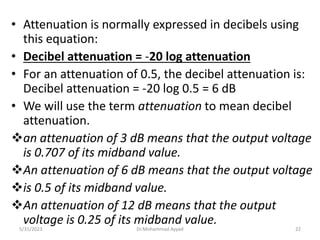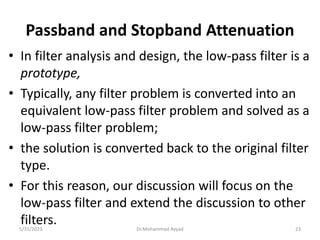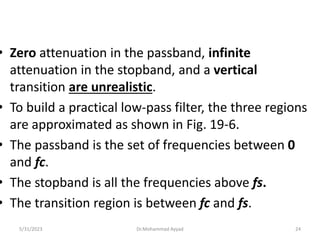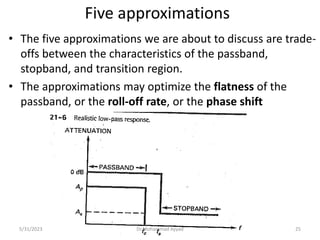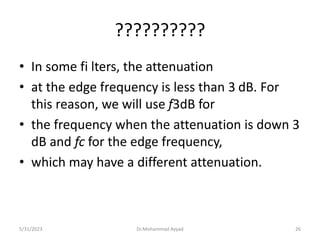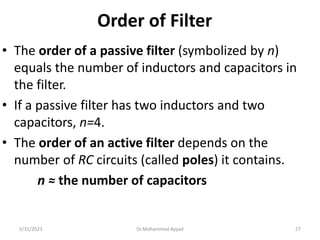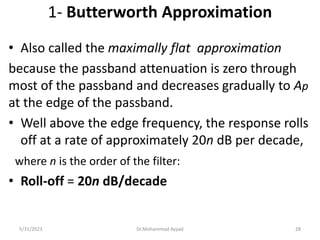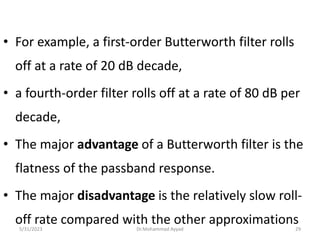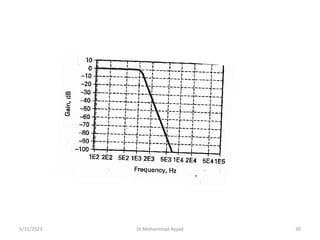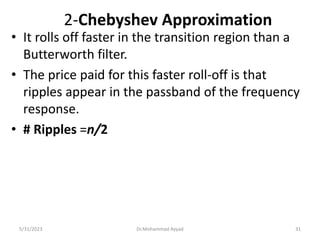1 de 31

### ACTIVE_FILTERS.pptx

• 1. Lecture 11 Chapter 15 ACTIVE FILTERS BASIC FILTER RESPONSES 5/31/2023 Dr.Mohammad Ayyad 1
• 2. INTRODUCTION • The frequency response of a filter is the graph of its voltage gain versus frequency. • A filter is a circuit that passes certain frequencies and attenuates or rejects all other frequencies. • The passband of a filter is the range of frequencies that are allowed to pass through the filter with minimum attenuation. (usually defined as less than – 3 dB of attenuation). 5/31/2023 Dr.Mohammad Ayyad 2
• 3. • The critical frequency, fc ,(also called the cutoff frequency) is defined as the end of the passband and is normally specified at the point where the response drops – 3 dB (70.7%) from the passband response. • Following the passband is a region called the transition region that leads into a region called the stopband. • There is no precise point between the transition region and the stopband. 5/31/2023 Dr.Mohammad Ayyad 3
• 4. Low-Pass Filter Response • A low-pass filter is one that passes frequencies from dc to fc , and significantly attenuates all other frequencies. • The passband of the ideal low-pass filter is shown in the blue shaded area of Figure 15–1(a); • The bandwidth of an ideal low-pass filter is equal to fc BW = fc 5/31/2023 Dr.Mohammad Ayyad 4
• 5. A decade of frequency change is a ten-times change (increase or decrease). 15–1(a); 5/31/2023 Dr.Mohammad Ayyad 5
• 7. Poles • A single RC circuit is called a pole.(In filter terminology). • Actual filter responses depend on the number of poles. • The -20 dB/decade roll-off rate for the gain of a basic RC filter means that at a frequency of 10fc,the output will be -20 dB (10%) of the input. 5/31/2023 Dr.Mohammad Ayyad 7
• 8. • The critical frequency of a low-pass RC filter occurs when XC = R, where fc =1/2πRC • The output at the critical frequency is 70.7% of the input. • This response is equivalent to an attenuation of -3 dB 5/31/2023 Dr.Mohammad Ayyad 8
• 10. Active filters • Filters that include one or more op-amps in the design are called active filters. • by combining an op-amp with frequency-selective feedback circuits, these filters can optimize: 1. the roll-off rate or 2. phase response • In general, the more poles the filter uses, the steeper its transition region will be 5/31/2023 Dr.Mohammad Ayyad 10
• 11. Order of Filter • The order of a passive filter (symbolized by n) equals the number of inductors and capacitors in the filter. • If a passive filter has two inductors and two capacitors, n=4. • The order of an active filter depends on the number of RC circuits (called poles) it contains. n ≈ the number of capacitors 5/31/2023 Dr.Mohammad Ayyad 11
• 14. Band-Pass Filter Response • A band-pass filter passes all signals lying within a band between a lower-frequency limit and an upper- frequency limit and essentially rejects all other frequencies that are outside this specified band. Bandwidth BW = fc2 - fc1 center frequency F0 = 5/31/2023 Dr.Mohammad Ayyad 14
• 15. Quality Factor (Q) • The frequency about which the passband is centered is called the center frequency, f0, defined as the geometric mean of the critical frequencies. • Quality Factor (Q) of a band-pass filter is the ratio of the center frequency to the bandwidth. • Q=f0/BW 5/31/2023 Dr.Mohammad Ayyad 15
• 16. • The value of Q is an indication of the selectivity of a band-pass filter. • The higher the value of Q, the narrower the bandwidth and the better the selectivity for a given value of f0. • Band-pass filters are sometimes classified as narrow-band (Q > 10)or wide-band(Q < 10). 5/31/2023 Dr.Mohammad Ayyad 16
• 17. EXAMPLE 15–1 • A certain band-pass filter has a center frequency of 15 kHz and a bandwidth of 1 kHz. Determine Q and classify the filter as narrow-band or wide-band 5/31/2023 Dr.Mohammad Ayyad 17
• 18. Band-Stop Filter Response also known as notch, band-reject, or band- elimination filter. 5/31/2023 Dr.Mohammad Ayyad 18
• 19. All-Pass Filter • The all-pass filter is useful when we want to produce a certain amount of phase shift for the signal being filtered without changing its amplitude. • The phase response of a filter is defined as the graph of phase shift versus frequency. 5/31/2023 Dr.Mohammad Ayyad 19
• 21. Approximate Responses • Attenuation • Attenuation refers to a loss of signal. • With a constant input voltage, attenuation is defined as the output voltage at any frequency divided by the output voltage in the midband: Attenuation =vout/vout(mid) • if the output voltage is 1 V and the output voltage in the midband is 2 V, then: • Attenuation = 1 V/ 2 V = 0.5 5/31/2023 Dr.Mohammad Ayyad 21
• 22. • Attenuation is normally expressed in decibels using this equation: • Decibel attenuation = -20 log attenuation • For an attenuation of 0.5, the decibel attenuation is: Decibel attenuation = -20 log 0.5 = 6 dB • We will use the term attenuation to mean decibel attenuation. an attenuation of 3 dB means that the output voltage is 0.707 of its midband value. An attenuation of 6 dB means that the output voltage is 0.5 of its midband value. An attenuation of 12 dB means that the output voltage is 0.25 of its midband value. 5/31/2023 Dr.Mohammad Ayyad 22
• 23. Passband and Stopband Attenuation • In filter analysis and design, the low-pass filter is a prototype, • Typically, any filter problem is converted into an equivalent low-pass filter problem and solved as a low-pass filter problem; • the solution is converted back to the original filter type. • For this reason, our discussion will focus on the low-pass filter and extend the discussion to other filters. 5/31/2023 Dr.Mohammad Ayyad 23
• 24. • Zero attenuation in the passband, infinite attenuation in the stopband, and a vertical transition are unrealistic. • To build a practical low-pass filter, the three regions are approximated as shown in Fig. 19-6. • The passband is the set of frequencies between 0 and fc. • The stopband is all the frequencies above fs. • The transition region is between fc and fs. 5/31/2023 Dr.Mohammad Ayyad 24
• 25. Five approximations • The five approximations we are about to discuss are trade- offs between the characteristics of the passband, stopband, and transition region. • The approximations may optimize the flatness of the passband, or the roll-off rate, or the phase shift 5/31/2023 Dr.Mohammad Ayyad 25
• 26. ?????????? • In some fi lters, the attenuation • at the edge frequency is less than 3 dB. For this reason, we will use f3dB for • the frequency when the attenuation is down 3 dB and fc for the edge frequency, • which may have a different attenuation. 5/31/2023 Dr.Mohammad Ayyad 26
• 27. Order of Filter • The order of a passive filter (symbolized by n) equals the number of inductors and capacitors in the filter. • If a passive filter has two inductors and two capacitors, n=4. • The order of an active filter depends on the number of RC circuits (called poles) it contains. n ≈ the number of capacitors 5/31/2023 Dr.Mohammad Ayyad 27
• 28. 1- Butterworth Approximation • Also called the maximally flat approximation because the passband attenuation is zero through most of the passband and decreases gradually to Ap at the edge of the passband. • Well above the edge frequency, the response rolls off at a rate of approximately 20n dB per decade, where n is the order of the filter: • Roll-off = 20n dB/decade 5/31/2023 Dr.Mohammad Ayyad 28
• 29. • For example, a first-order Butterworth filter rolls off at a rate of 20 dB decade, • a fourth-order filter rolls off at a rate of 80 dB per decade, • The major advantage of a Butterworth filter is the flatness of the passband response. • The major disadvantage is the relatively slow roll- off rate compared with the other approximations 5/31/2023 Dr.Mohammad Ayyad 29
• 31. 2-Chebyshev Approximation • It rolls off faster in the transition region than a Butterworth filter. • The price paid for this faster roll-off is that ripples appear in the passband of the frequency response. • # Ripples =n/2 5/31/2023 Dr.Mohammad Ayyad 31